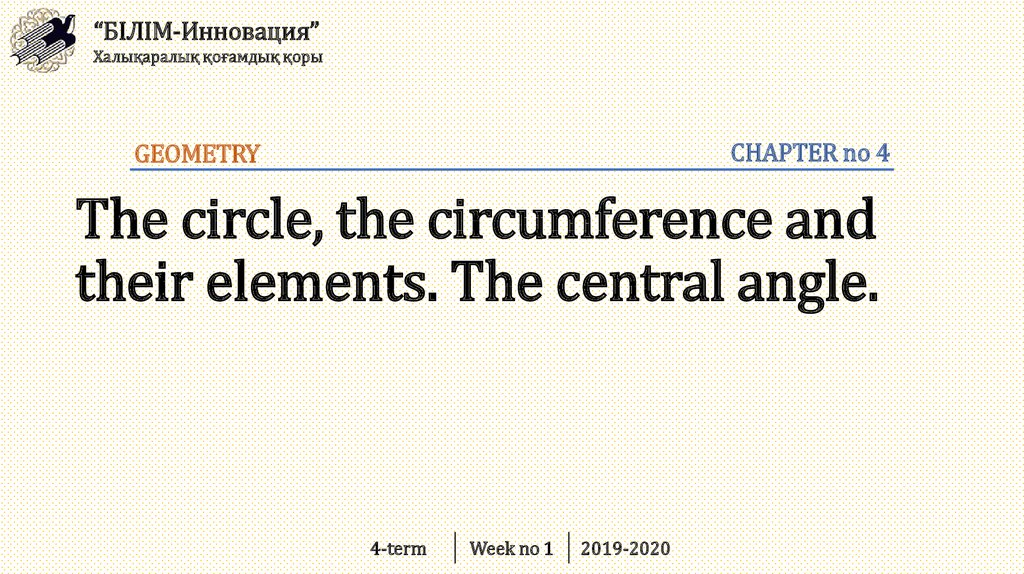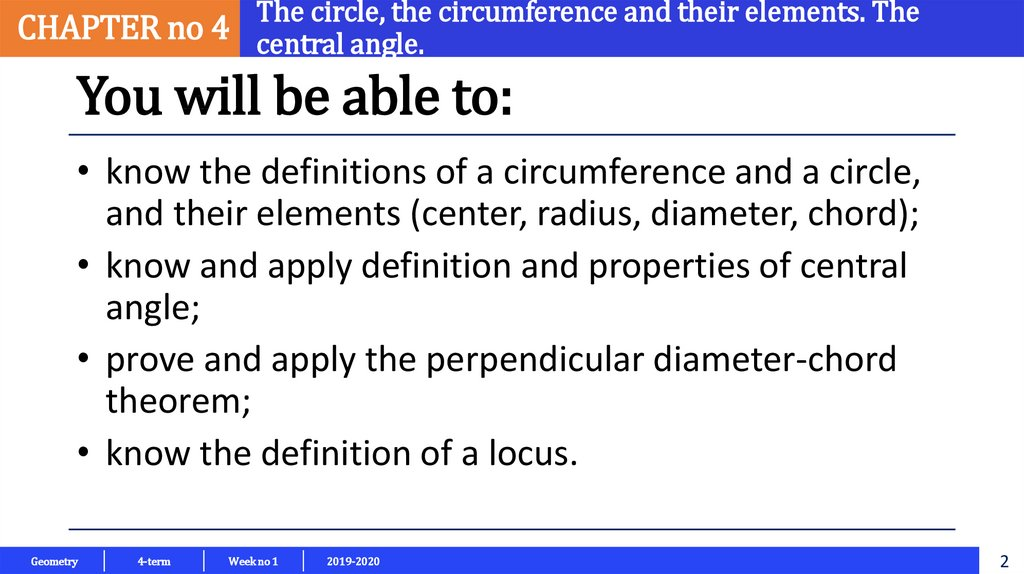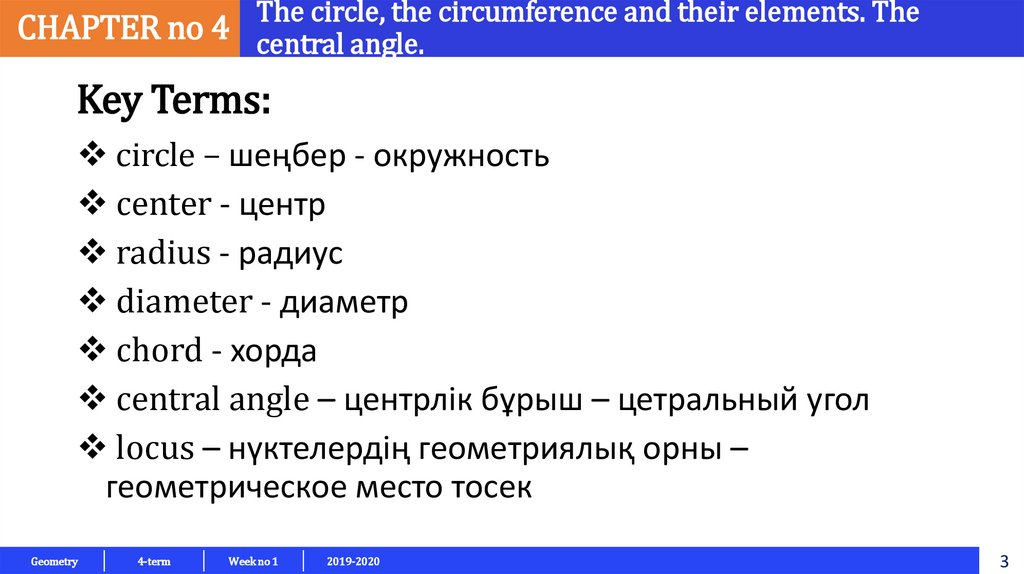# The circle, the circumference and their elements. The central angle

## 1. The circle, the circumference and their elements. The central angle.

“БІЛІМ-Инновация”
Халықаралық қоғамдық қоры
CHAPTER no 4
GEOMETRY
The circle, the circumference and
their elements. The central angle.
4-term
Week no 1
2019-2020

## 2.

The circle, the circumference and their elements. The
CHAPTER no 4 central angle.
You will be able to:
• know the definitions of a circumference and a circle,
and their elements (center, radius, diameter, chord);
• know and apply definition and properties of central
angle;
• prove and apply the perpendicular diameter-chord
theorem;
• know the definition of a locus.
Geometry
4-term
Week no 1
2019-2020
2

## 3.

The circle, the circumference and their elements. The
CHAPTER no 4 central angle.
Key Terms:
circle – шеңбер - окружность
center - центр
diameter - диаметр
chord - хорда
central angle – центрлік бұрыш – цетральный угол
locus – нүктелердің геометриялық орны –
геометрическое место тосек
Geometry
4-term
Week no 1
2019-2020
3

## 4.

The circle, the circumference and their elements. The
CHAPTER no 4 central angle.
You can see many circular or ring-shaped geometric figures all
around you.
Geometry
4-term
Week no 1
2019-2020
4

## 5.

The circle, the circumference and their elements. The
CHAPTER no 4 central angle.
It is easy to recognize a circle, but
how can we define it as a shape?
Let us look at a geometric
definition.
Note:
The word ‘circle’ is
derived from the latin
word circus, which
means ‘ring’ or
‘racecourse’.
Geometry
4-term
Week no 1
2019-2020
5

## 6.

The circle, the circumference and their elements. The
CHAPTER no 4 central angle.
Definition:
A CIRCLE is the set of all the points in a
plane that are at the same distance from a
fixed point in the plane and the fixed point
is called the center of the circle.
Geometry
4-term
Week no 1
2019-2020
6

## 7.

The circle, the circumference and their elements. The
CHAPTER no 4 central angle.
Definition:
The RADIUS is the distance from the center
The DIAMETER goes straight across the circle,
through the center
The CHORD is a line segment which
joins two different points on a circle.
Geometry
4-term
Week no 1
2019-2020
7

## 8.

The circle, the circumference and their elements. The
CHAPTER no 4 central angle.
Definition:
Circumference is the distance once around the circle
Circumference = π × Diameter
Geometry
4-term
Week no 1
2019-2020
8

## 9.

The circle, the circumference and their elements. The
CHAPTER no 4 central angle.
Example:
You walk around a circle which has a
diameter of 100m, how far have you
walked?
Solution:
Distance walked = Circumference = π × 100m
= 314m (to the nearest m)
Geometry
4-term
Week no 1
2019-2020
9

## 10.

The circle, the circumference and their elements. The
CHAPTER no 4 central angle.
Example:
The length of the diameter of a circle is 20 cm and
the radius is 2x – 4. Find x.
Solution:
d=2⋅r
20 = 2 ⋅ (2x – 4)
2x – 4 = 10
2x = 14
x = 7 cm
Geometry
4-term
Week no 1
2019-2020
10

## 11.

The circle, the circumference and their elements. The
CHAPTER no 4 central angle.
Definition:
An ARC of a circle consists of two points on the circle and the
unbroken part of the circle between these two points.
NOTE:
Any two points of a circle divide the
circle into two arcs. If the arcs are
unequal, the smaller arc is called the
minor arc and the larger arc is called
the major arc.
Geometry
4-term
Week no 1
2019-2020
11

## 12.

The circle, the circumference and their elements. The
CHAPTER no 4 central angle.
Definition:
A CENTRAL ANGLE of the circle is an angle
whose vertex is at the center of a circle.
NOTE:
The measure of a minor arc
is equal to the measure of
its central angle.
Geometry
4-term
Week no 1
2019-2020
Central Angle = Minor Arc
∠APB = A͡B
12

## 13.

The circle, the circumference and their elements. The
CHAPTER no 4 central angle.
Examples:
Find the measure of the indicated central angle of each
circle.
Geometry
4-term
Week no 1
2019-2020
13

## 14.

The circle, the circumference and their elements. The
CHAPTER no 4 central angle.
Theorem:
If a diameter or radius is perpendicular to a chord,
then it bisects the chord and its arc.
Example:
In the figure, if [OH] ⊥ [AB] then
|AH| = |HB|.
Geometry
4-term
Week no 1
2019-2020

## 15.

The circle, the circumference and their elements. The
CHAPTER no 4 central angle.
Example:
A chord of length 10 cm is 12 cm away from the center of a
circle. Find the length of the radius.
Solution:
Look at the figure.
In ΔAHO, r² = 5² + 12²
r² = 25 + 144
r² = 169
r = 13 cm.
Geometry
4-term
Week no 1
2019-2020

## 16.

The circle, the circumference and their elements. The
CHAPTER no 4 central angle.
Definition:
LOCUS is the set of all points that share a property. This usually results in a curve
or surface.
Example:
A Circle is "the locus of points on a plane that are a certain distance from a central
point".
Geometry
4-term
Week no 1
2019-2020

## 17.

The circle, the circumference and their elements. The
CHAPTER no 4 central angle.
Activity:
Put a pin in a board, put a loop of string around it,
and insert a pencil into the loop. Keep the string
stretched and draw the circle!
Geometry
4-term
Week no 1
2019-2020

## 18.

The circle, the circumference and their elements. The
CHAPTER no 4 central angle.
Resources :
https://www.mathsisfun.com/geometry/circle.html
https://www.onlinemathlearning.com/diameter-of-circle.html
https://www.mathemania.com/lesson/the-definition-and-basics/